# Game Math: Swing-Twist Interpolation (…Sterp?)

This post is part of my Game Math Series.

Source files are on GitHub.
Shortcut to sterp implementation.
Shortcut to code used to generate animations in this post.

### An Alternative to Slerp

Slerp, spherical linear interpolation, is an operation that interpolates from one orientation to another, using a rotational axis paired with the smallest angle possible.

Quick note: Jonathan Blow explains here how you should avoid using slerp, if normalized quaternion linear interpolation (nlerp) suffices. Long store short, nlerp is faster but does not maintain constant angular velocity, while slerp is slower but maintains constant angular velocity; use nlerp if you’re interpolating across small angles or you don’t care about constant angular velocity; use slerp if you’re interpolating across large angles and you care about constant angular velocity. But for the sake of using a more commonly known and used building block, the remaining post will only mention slerp. Replacing all following occurrences of slerp with nlerp would not change the validity of this post.

In general, slerp is considered superior over interpolating individual components of Euler angles, as the latter method usually yields orientational sways.

But, sometimes slerp might not be ideal. Look at the image below showing two different orientations of a rod. On the left is one orientation, and on the right is the resulting orientation of rotating around the axis shown as a cyan arrow, where the pivot is at one end of the rod.If we slerp between the two orientations, this is what we get: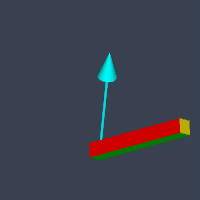Mathematically, slerp takes the “shortest rotational path”. The quaternion representing the rod’s orientation travels along the shortest arc on a 4D hypersphere. But, given the rod’s elongated appearance, the rod’s moving end seems to be deviating from the shortest arc on a 3D sphere.

My intended effect here is for the rod’s moving end to travel along the shortest arc in 3D, like this: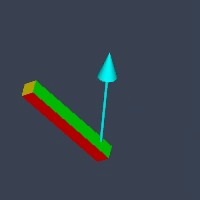The difference is more obvious if we compare them side-by-side: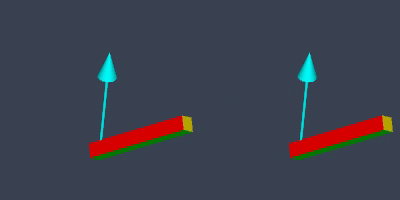This is where swing-twist decomposition comes in.

### Swing-Twist Decomposition

Swing-Twist decomposition is an operation that splits a rotation into two concatenated rotations, swing and twist. Given a twist axis, we would like to separate out the portion of a rotation that contributes to the twist around this axis, and what’s left behind is the remaining swing portion.

There are multiple ways to derive the formulas, but this particular one by Michaele Norel seems to be the most elegant and efficient, and it’s the only one I’ve come across that does not involve any use of trigonometry functions. I will first show the formulas now and then paraphrase his proof later:

Given a rotation represented by a quaternion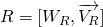and a twist axis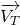, combine the scalar part fromthe projection of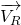ontoto form a new quaternion:We want to decomposeinto a swing component and a twist component. Let the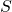denote the swing component, so we can write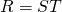. The swing component is then calculated by multiplyingwith the inverse (conjugate) of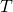: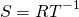Beware thatandare not yet normalized at this point. It’s a good idea to normalize them before use, as unit quaternions are just cuter.

Below is my code implementation of swing-twist decomposition. Note that it also takes care of the singularity that occurs when the rotation to be decomposed represents a 180-degree rotation.

public static void DecomposeSwingTwist
(
Quaternion q,
Vector3 twistAxis,
out Quaternion swing,
out Quaternion twist
)
{
Vector3 r = new Vector3(q.x, q.y, q.z);

// singularity: rotation by 180 degree
if (r.sqrMagnitude < MathUtil.Epsilon)
{
Vector3 rotatedTwistAxis = q * twistAxis;
Vector3 swingAxis =
Vector3.Cross(twistAxis, rotatedTwistAxis);

if (swingAxis.sqrMagnitude > MathUtil.Epsilon)
{
float swingAngle =
Vector3.Angle(twistAxis, rotatedTwistAxis);
swing = Quaternion.AngleAxis(swingAngle, swingAxis);
}
else
{
// more singularity:
// rotation axis parallel to twist axis
swing = Quaternion.identity; // no swing
}

// always twist 180 degree on singularity
twist = Quaternion.AngleAxis(180.0f, twistAxis);
return;
}

// meat of swing-twist decomposition
Vector3 p = Vector3.Project(r, twistAxis);
twist = new Quaternion(p.x, p.y, p.z, q.w);
twist = Normalize(twist);
swing = q * Quaternion.Inverse(twist);
}


Now that we have the means to decompose a rotation into swing and twist components, we need a way to use them to interpolate the rod’s orientation, replacing slerp.

### Swing-Twist Interpolation

Replacing slerp with the swing and twist components is actually pretty straightforward. Let the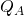and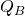denote the quaternions representing the rod’s two orientations we are interpolating between. Given the interpolation parameter, we use it to find “fractions” of swing and twist components and combine them together. Such fractiona can be obtained by performing slerp from the identity quaternion,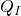, to the individual components.

So we replace: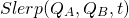with: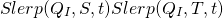From the rod example, we choose the twist axis to align with the rod’s longest side. Let’s look at the effect of the individual components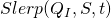andasvaries over time below, swing on left and twist on right: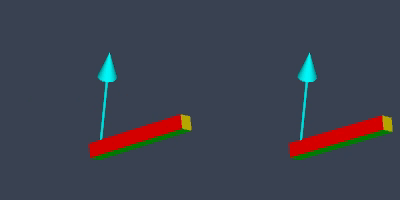And as we concatenate these two components together, we get a swing-twist interpolation that rotates the rod such that its moving end travels in the shortest arc in 3D. Again, here is a side-by-side comparison of slerp (left) and swing-twist interpolation (right):I decided to name my swing-twist interpolation function sterp. I think it’s cool because it sounds like it belongs to the function family of lerp and slerp. Here’s to hoping that this name catches on.

And here’s my code implementation:

public static Quaternion Sterp
(
Quaternion a,
Quaternion b,
Vector3 twistAxis,
float t
)
{
Quaternion deltaRotation = b * Quaternion.Inverse(a);

Quaternion swingFull;
Quaternion twistFull;
QuaternionUtil.DecomposeSwingTwist
(
deltaRotation,
twistAxis,
out swingFull,
out twistFull
);

Quaternion swing =
Quaternion.Slerp(Quaternion.identity, swingFull, t);
Quaternion twist =
Quaternion.Slerp(Quaternion.identity, twistFull, t);

return twist * swing;
}


### Proof

Lastly, let’s look at the proof for the swing-twist decomposition formulas. All that needs to be proven is that the swing componentdoes not contribute to any rotation around the twist axis, i.e. the rotational axis ofis orthogonal to the twist axis.

Let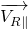denote the parallel component ofto, which can be obtained by projectingonto: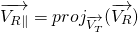Let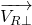denote the orthogonal component ofto: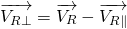So the scalar-vector form ofbecomes:Using the quaternion multiplication formula, here is the scalar-vector form of the swing quaternion: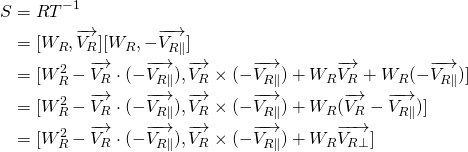Take notice of the vector part of the result: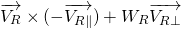This is a vector parallel to the rotational axis of. Both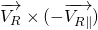andare orthogonal to the twist axis, so we have shown that the rotational axis ofis orthogonal to the twist axis. Hence, we have proven that the formulas forandare valid for swing-twist decomposition.

### Conclusion

That’s all.

Given a twist axis, I have shown how to decompose a rotation into a swing component and a twist component.

Such decomposition can be used for swing-twist interpolation, an alternative to slerp that interpolates between two orientations, which can be useful if you’d like some point on a rotating object to travel along the shortest arc.

I like to call such interpolation sterp.

Sterp is merely an alternative to slerp, not a replacement. Also, slerp is definitely more efficient than sterp. Most of the time slerp should work just fine, but if you find unwanted orientational sway on an object’s moving end, you might want to give sterp a try.

### Edit: Application in 2D

An application of swing-twist decomposition in 2D just came to mind.

If the twist axis is chosen to be orthogonal to the screen, then we can utilize swing-twist decomposition to use the orientation of objects in 3D to drive the rotation of 2D elements in screen space or some other data. The twist component represents exactly the portion of 3D rotation projected onto screen space.

However, in terms of performance, we might be better off just projecting a 3D object’s local axis onto screen space and find the angle between it and a screen space axis. But then again, the swing-twist decomposition approach doesn’t have the singularity the projection approach has when the chosen local axis becomes orthogonal to the screen.Physics / Graphics / Procedural Animation / Visuals
This entry was posted in Gamedev, Math, Programming, Unity. Bookmark the permalink.

### 1 Response to Game Math: Swing-Twist Interpolation (…Sterp?)

1.fxc says:

Hi,

I think you need to multiply by the result by a in your sterp function, ie. twist * swing * a. Because twist * swing is only the delta rotation. If we want a quaternion between a and b, we need to start from a, and as such multiply by a in the result.

Thanks for the interesting article!

This site uses Akismet to reduce spam. Learn how your comment data is processed.